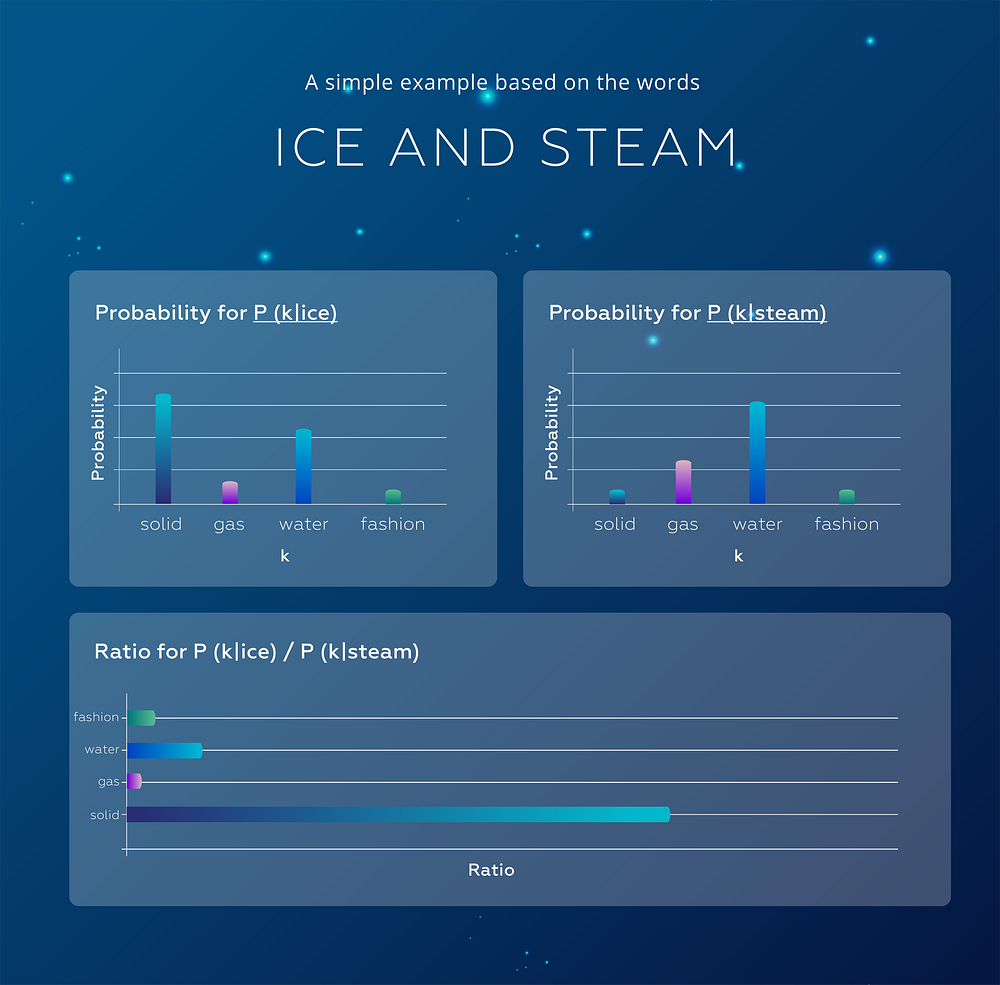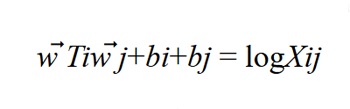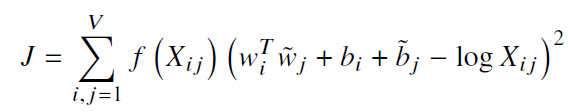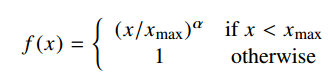# Word Vectors in Natural Language Processing: Global Vectors (GloVe)

A well-known model that learns vectors or words from their co-occurrence information is GlobalVectors (GloVe). While word2vec is a predictive model — a feed-forward neural network that learns vectors to improve the predictive ability, GloVe is a count-based model.comments

By Sciforce.Another well-known model that learns vectors or words from their co-occurrence information, i.e. how frequently they appear together in large text corpora, is GlobalVectors (GloVe). While word2vec is a predictive model — a feed-forward neural network that learns vectors to improve the predictive ability, GloVe is a count-based model.

### What is a count-based model?

Generally speaking, count-based models learn vectors by doing dimensionality reduction on a co-occurrence counts matrix. First they construct a large matrix of co-occurrence information, which contains the information on how frequently each “word” (stored in rows), is seen in some “context” (the columns). The number of “contexts” needs be large, since it is essentially combinatorial in size. Afterwards they factorize this matrix to yield a lower-dimensional matrix of words and features, where each row yields a vector representation for each word. It is achieved by minimizing a “reconstruction loss” which looks for lower-dimensional representations that can explain the variance in the high-dimensional data.

In the case of GloVe, the counts matrix is preprocessed by normalizing the counts and log-smoothing them. Compared to word2vec, GloVe allows for parallel implementation, which means that it’s easier to train over more data. It is believed (GloVe) to combine the benefits of the word2vec skip-gram model in the word analogy tasks, with those of matrix factorization methods exploiting global statistical information.

### GloVe at a Glance

On the project page it is stated that GloVe is essentially a log-bilinear model with a weighted least-squares objective. The model rests on a rather simple idea that ratios of word-word co-occurrence probabilities have the potential for encoding some form of meaning which can be encoded as vector differences. Therefore, the training objective is to learn word vectors such that their dot product equals the logarithm of the words’ probability of co-occurrence. As the logarithm of a ratio equals the difference of logarithms, this objective associates the ratios of co-occurrence probabilities with vector differences in the word vector space. It creates the word vectors that perform well on both word analogy tasks and on similarity tasks and named entity recognition.

### Finding meaning in statistics with GloVe

Almost all unsupervised methods for learning word representations use the statistics of word occurrences in a corpus as the primary source of information, yet the question remains as to how we can generate meaning from these statistics, and how the resulting word vectors might represent that meaning.

Pennington et al. (2014) present a simple example based on the words ice and steam to illustrate it.

The relationship of these words can be revealed by studying the ratio of their co-occurrence probabilities with various probe words, k. Let P(k|w) be the probability that the word k appears in the context of word w: ice co-occurs more frequently with solid than it does with gas, whereas steam co-occurs more frequently with gas than it does with solid. Both words co-occur frequently with water (as it is their shared property ) and infrequently — with the unrelated word fashion.

In other words, P(solid | ice) will be relatively high, and P(solid | steam) will be relatively low. Therefore, the ratio of P(solid | ice) / P(solid | steam) will be large. If we take a word such as gas that is related to steam but not to ice, the ratio of P(gas | ice) / P(gas | steam) will instead be small. For a word related to both ice and steam, such as water we expect the ratio to be close to one:We can see that the appropriate starting point for word vector learning might be indeed with ratios of co-occurrence probabilities rather than the probabilities themselves.

### Cost Function

The way GloVe predicts surrounding words is by maximizing the probability of a context word occurring given a center word by performing a dynamic logistic regression.

Before training the actual model, a co-occurrence matrix X is constructed, where a cell Xij is a “strength” which represents how often the word i appears in the context of the word j. Once X is ready, it is necessary to decide vector values in continuous space for each word in the corpus, in other words, to build word vectors that show how every pair of words i and j co-occur.

We will produce vectors with a soft constraint that for each word pair of word i and word jwhere bi and bj are scalar bias terms associated with words i and j, respectively.

We’ll do this by minimizing an objective function J, which evaluates the sum of all squared errors based on the above equation, weighted with a function f:Where V is the size of the vocabulary.

Yet, some co-occurrences that happen rarely or never are noisy and carry less information than the more frequent ones. To deal with them, a weighted least squares regression model is used. One class of weighting functions found to work well can be parametrized asThe model generates two sets of word vectors,W and W̃. When X is symmetric,W and W̃ are equivalent and differ only as a result of their random initializations; the two sets of vectors should perform equivalently. As for certain types of neural networks, training multiple instances of the network and then combining the results can help reduce overfitting and noise (Ciresan et al., 2012), W and W̃ are summed up as word vectors. Doing so gives a small boost in performance, with the biggest increase in the semantic analogy task.

### Results

The model utilizes the main benefit of count data — the ability to capture global statistics — while simultaneously capturing the meaningful linear substructures prevalent in recent log-bilinear prediction-based methods like word2vec. As a result, GloVe becomes a global log-bilinear regression model for the unsupervised learning of word representations that outperforms other models on word analogy, word similarity, and named entity recognition tasks.

• Fast training
• Scalable to huge corpora
• Good performance even with small corpus, and small vectors
• Early stopping. We can stop training when improvements become small.

#### Drawbacks

• Uses a lot of memory: the fastest way to construct a term co-occurence matrix is to keep it in RAM as a hash map and perform co-occurence increments in a global manner
• Sometimes quite sensitive to initial learning rate

The source code for the model, as well as trained word vectors can be found at the project page at http://nlp.stanford.edu/projects/glove/

Original. Reposted with permission.

About: Sciforce is a Ukraine-based IT company developing software solutions, with a wide-ranging expertise in key AI technologies, including Data Mining, Digital Signal Processing, Natural Language Processing, Machine Learning, Image Processing and Computer Vision.

Related: